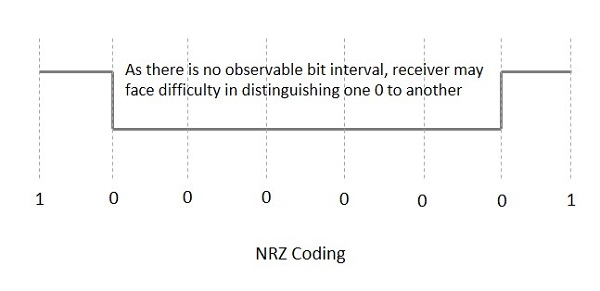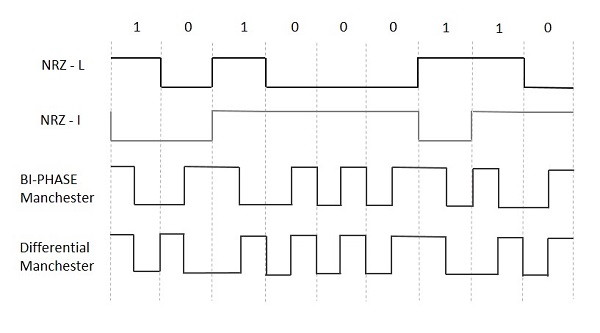# Data Encoding Techniques

Encoding is the process of converting the data or a given sequence of characters, symbols, alphabets etc., into a specified format, for the secured transmission of data. Decoding is the reverse process of encoding which is to extract the information from the converted format.

### Data Encoding

Encoding is the process of using various patterns of voltage or current levels to represent 1s and 0s of the digital signals on the transmission link.

The common types of line encoding are Unipolar, Polar, Bipolar, and Manchester.

## Encoding Techniques

The data encoding technique is divided into the following types, depending upon the type of data conversion.

• Analog data to Analog signals − The modulation techniques such as Amplitude Modulation, Frequency Modulation and Phase Modulation of analog signals, fall under this category.

• Analog data to Digital signals − This process can be termed as digitization, which is done by Pulse Code Modulation (PCM). Hence, it is nothing but digital modulation. As we have already discussed, sampling and quantization are the important factors in this. Delta Modulation gives a better output than PCM.

• Digital data to Analog signals − The modulation techniques such as Amplitude Shift Keying (ASK), Frequency Shift Keying (FSK), Phase Shift Keying (PSK), etc., fall under this category. These will be discussed in subsequent chapters.

• Digital data to Digital signals − These are in this section. There are several ways to map digital data to digital signals. Some of them are −

NRZ Codes has 1 for High voltage level and 0 for Low voltage level. The main behavior of NRZ codes is that the voltage level remains constant during bit interval. The end or start of a bit will not be indicated and it will maintain the same voltage state, if the value of the previous bit and the value of the present bit are same.

The following figure explains the concept of NRZ coding.If the above example is considered, as there is a long sequence of constant voltage level and the clock synchronization may be lost due to the absence of bit interval, it becomes difficult for the receiver to differentiate between 0 and 1.

There are two variations in NRZ namely −

### NRZ - L (NRZ – LEVEL)

There is a change in the polarity of the signal, only when the incoming signal changes from 1 to 0 or from 0 to 1. It is the same as NRZ, however, the first bit of the input signal should have a change of polarity.

### NRZ - I (NRZ – INVERTED)

If a 1 occurs at the incoming signal, then there occurs a transition at the beginning of the bit interval. For a 0 at the incoming signal, there is no transition at the beginning of the bit interval.

NRZ codes has a disadvantage that the synchronization of the transmitter clock with the receiver clock gets completely disturbed, when there is a string of 1s and 0s. Hence, a separate clock line needs to be provided.

## Bi-phase Encoding

The signal level is checked twice for every bit time, both initially and in the middle. Hence, the clock rate is double the data transfer rate and thus the modulation rate is also doubled. The clock is taken from the signal itself. The bandwidth required for this coding is greater.

There are two types of Bi-phase Encoding.

• Bi-phase Manchester
• Differential Manchester

### Bi-phase Manchester

In this type of coding, the transition is done at the middle of the bit-interval. The transition for the resultant pulse is from High to Low in the middle of the interval, for the input bit 1. While the transition is from Low to High for the input bit 0.

### Differential Manchester

In this type of coding, there always occurs a transition in the middle of the bit interval. If there occurs a transition at the beginning of the bit interval, then the input bit is 0. If no transition occurs at the beginning of the bit interval, then the input bit is 1.

The following figure illustrates the waveforms of NRZ-L, NRZ-I, Bi-phase Manchester and Differential Manchester coding for different digital inputs.## Block Coding

Among the types of block coding, the famous ones are 4B/5B encoding and 8B/6T encoding. The number of bits are processed in different manners, in both of these processes.

### 4B/5B Encoding

In Manchester encoding, to send the data, the clocks with double speed is required rather than NRZ coding. Here, as the name implies, 4 bits of code is mapped with 5 bits, with a minimum number of 1 bits in the group.

The clock synchronization problem in NRZ-I encoding is avoided by assigning an equivalent word of 5 bits in the place of each block of 4 consecutive bits. These 5-bit words are predetermined in a dictionary.

The basic idea of selecting a 5-bit code is that, it should have one leading 0 and it should have no more than two trailing 0s. Hence, these words are chosen such that two transactions take place per block of bits.

### 8B/6T Encoding

We have used two voltage levels to send a single bit over a single signal. But if we use more than 3 voltage levels, we can send more bits per signal.

For example, if 6 voltage levels are used to represent 8 bits on a single signal, then such encoding is termed as 8B/6T encoding. Hence in this method, we have as many as 729 (3^6) combinations for signal and 256 (2^8) combinations for bits.

These are the techniques mostly used for converting digital data into digital signals by compressing or coding them for reliable transmission of data.